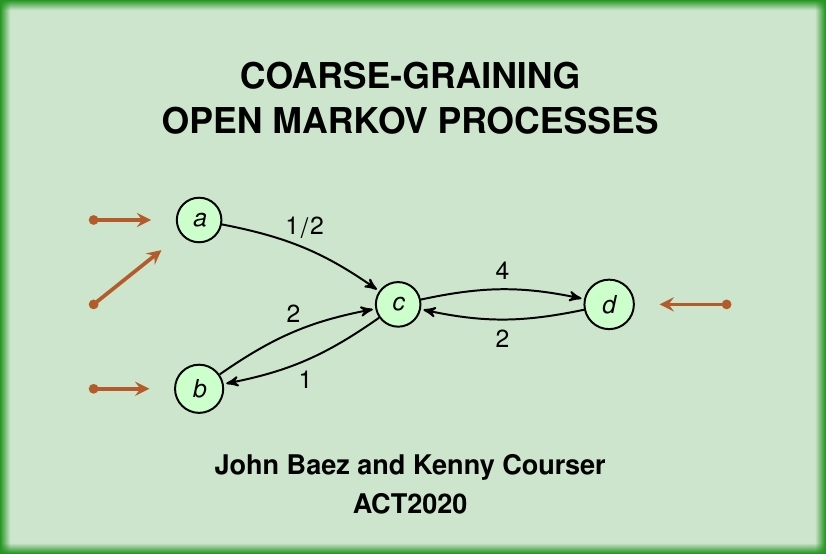#### July 7, 2020 — Applied Category Theory 2020## Coarse-Graining Open Markov Processes

We illustrate some new paradigms in applied category theory with the example of coarse-graining open Markov processes. Coarse-graining is a standard method of extracting a simpler Markov process from a more complicated one by identifying states. Here we extend coarse-graining to 'open' Markov processes: that is, those where probability can flow in or out of certain states called 'inputs' and 'outputs'. One can build up an ordinary Markov process from smaller open pieces in two basic ways: composition, where we identify the outputs of one open Markov process with the inputs of another, and tensoring, where we set two open Markov processes side by side. These constructions make open Markov processes into the morphisms of a symmetric monoidal category. But we can go further and construct a symmetric monoidal double category where the 2-morphisms include ways of coarse-graining open Markov processes. We can describe the behavior of open Markov processes using double functors out of this double category.

You can see the slides here or watch a video:

This talk is based on work with Kenny Courser and others:

All this is part of a bigger project: